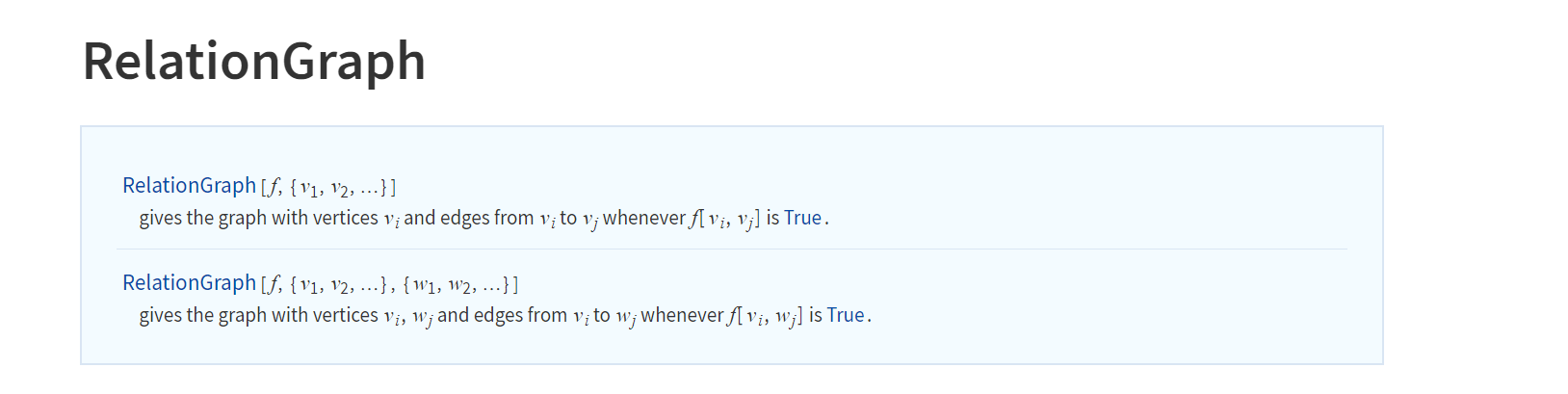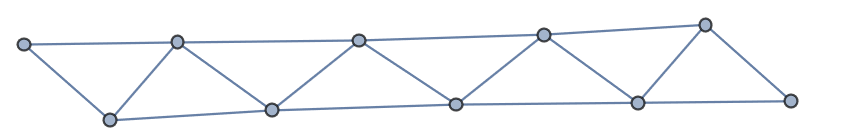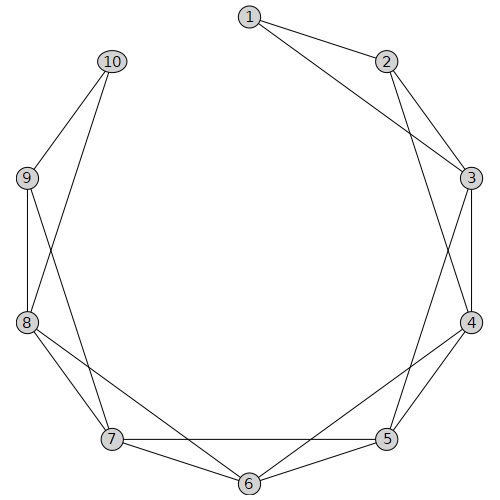# Question:Is there a maple function comparable to RelationGraph?

## Question:Is there a maple function comparable to RelationGraph?

Maple 2022

Recently, I tried to write a function to get the lexicographic product of two graphs.

In  graph theory the lexicographic product G ∙ H of graphs G and H is a graph such that
• the vertex set of G ∙ H is the cartesian product V(G) × V(H); and
• any two vertices (u,v) and (x,y) are adjacent in G ∙ H if and only if either u is adjacent with x in G or u = x and v is adjacent with y in H.

I'm trying to write this function as defined after making sure it doesn't exist in maple. I saw a similar post by mathematica stack.

There are many answers in the post, the most interesting to me is the following code, which follows the definition of lexicographic product.

``````lexicographicProduct[g1_?UndirectedGraphQ, g2_?UndirectedGraphQ, opt : OptionsPattern[]] :=
RelationGraph[
(* two nodes are connected if their corresponding nodes in the first graph are connected *)
EdgeQ[g1, First[#1] \[UndirectedEdge] First[#2]] ||
(* or their corresponding nodes in the first graph are the same and their corresponding nodes in the second graph are connected *)
(First[#1] === First[#2] && EdgeQ[g2, Last[#1] \[UndirectedEdge] Last[#2]]) &,

(* the vertices are the cartesian product of the two vertex sets *)
Tuples[{VertexList[g1], VertexList[g2]}],

(* also allow setting graph options *)
opt
]

lexicographicProduct[CycleGraph, CycleGraph]``````

It utilizes the  function `RelationGraph` in Mathematica. I feel that this function is generic in nature. So here I would ask maple if they had a similar function.

Function `RelationGraph` is to generate a graph based on data and a binary relation.For example, using `RelationGraph`  I  can get easily  the kth power Gk of an graph G which is another graph that has the same set of vertices, but in which two vertices are adjacent when their distance in G is at most k.

```Dis[g1_?UndirectedGraphQ, k_] :=
RelationGraph[
GraphDistance[g1, #1, #2] <= k && GraphDistance[g1, #1, #2] != 0 &,
VertexList[g1]]
Dis[PathGraph[Range], 2]
```If I use maple and do not use the built-in function `GraphPower`, I might deal with the following.

```with(GraphTheory):
with(SpecialGraphs):
graphpower:=proc(G,k):
local choo,edge,vex,g;
vex:=convert(Vertices(G),list);
choo:= choose(vex, 2):
g:= Graph(Vertices(G)):
for edge in choo do
if Distance(G, edge, edge)<=k  then
fi;
end do:
return g;
end proc:
s:=graphpower(PathGraph(10),2);DrawGraph(s)
```I believe if the `RelationGraph` function can be  implemented in maple, the function `lexicographicProduct` would be easier to obtain.﻿